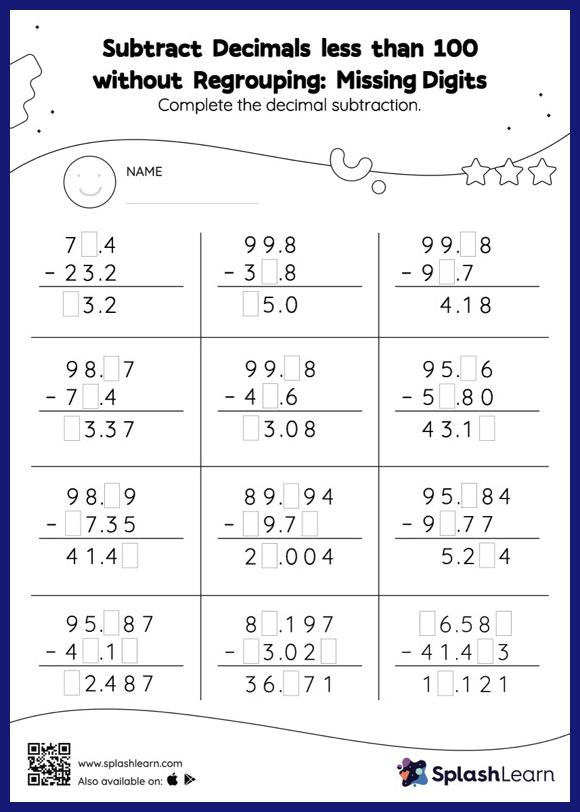# Subtract Decimals less than 100 without Regrouping: Missing Digits Worksheet

Home > Subtract Decimals less than 100 without Regrouping: Missing DigitsThis worksheet challenges young mathematicians to hone their math skills by solving a set of problems on subtracting decimals less than 100 without regrouping. Students find the missing number while subtracting decimals by using the relationship between addition and subtraction. Subtract decimals less than 100 without regrouping worksheet does not require them to regroup numbers.